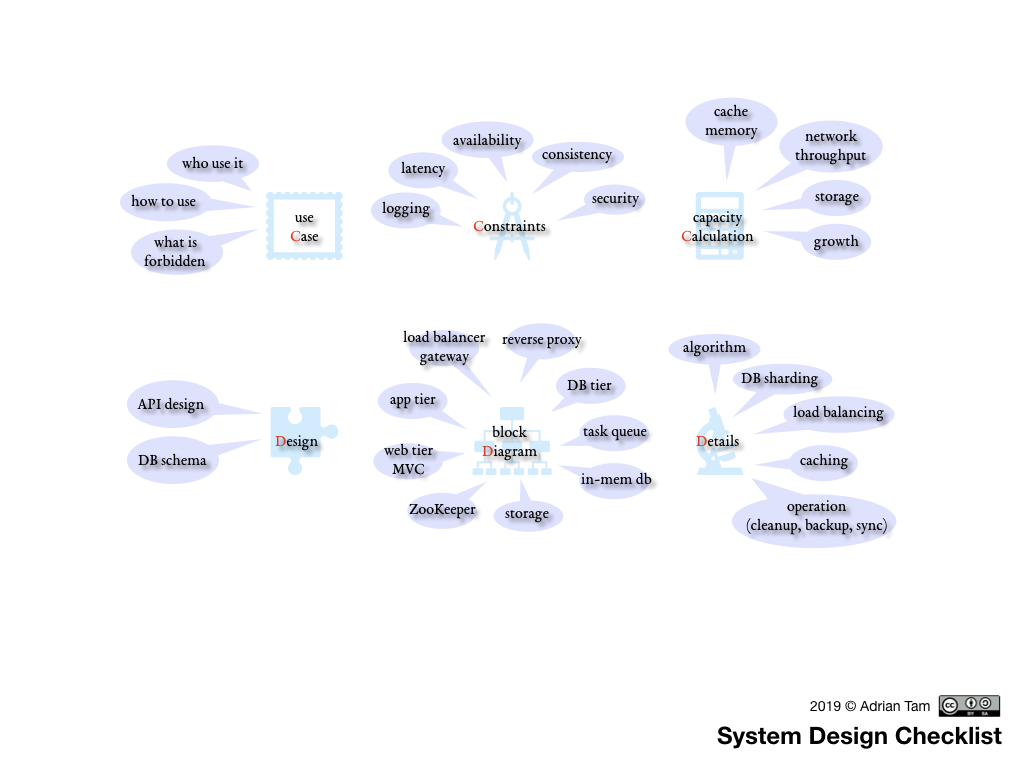This is a system design checklist I made to summarize a handful of videos I watched on the topic:But the point I want to make is to try out TikZJax, which claims to make the TikZ drawing system to work on the browser (WebAssembly required). It worked partially, and I have no idea how to debug:

The above is generated using the code below, which works fine in LaTeX command line but the relative coordinate system are treated as absolute coordinates in the WebAssembly version:

\begin{tikzpicture}

\draw (0,5) node[draw,circle,minimum size=2cm,align=center] (case) {use\\ \textcolor{red}{C}ase};
\node (case1) at ++(90:1cm) {who use it};
\node (case2) at ++(135:1cm) {how to use};
\node[align=center] (case3) at ++(180:1cm) {what is\\ forbidden};

\draw (5,5) node[draw,circle,minimum size=2cm] (constr) {\textcolor{red}{C}onstraints};
\node (constr1) at ++(180:1cm) {logging};
\node (constr2) at ++(135:1cm) {latency};
\node (constr3) at ++(90:1cm) {availability};
\node (constr4) at ++(45:1cm) {consistency};
\node (constr5) at ++(0:1cm) {security};

\draw (10,5) node[draw,circle,minimum size=2cm,align=center] (calcu) {capacity\\ \textcolor{red}{C}alculation};
\node[align=center] (calcu1) at ++(90:1cm) {cache\\ memory};
\node[align=center] (calcu2) at ++(45:1cm) {network\\ throughput};
\node (calcu3) at ++(0:1cm) {storage};
\node (calcu4) at ++(315:1cm) {growth};

\node[draw,circle,minimum size=2cm] (design) at (0,0) {\textcolor{red}{D}esign};
\node (design1) at ++(270:1cm) {API design};
\node (design2) at ++(225:1cm) {DB schema};

\node[draw,circle,minimum size=2cm,align=center] (diagram) at (5,0) {Block\\ \textcolor{red}{D}iagram};
\node (diagram1) at ++(0:20mm) {DB tier};
\node[align=center] (diagram2) at ++(72:20mm) {reverse\\ proxy};
\node (diagram7) at ++(36:20mm) {storage};
\node (diagram4) at ++(108:20mm) {app tier};
\node[align=center] (diagram5) at ++(144:20mm) {web tier\\ MVC};
\node (diagram6) at ++(180:20mm) {ZooKeeper};
\node[align=center] (diagram3) at ++(216:20mm) {load balancer\\ gateway};
\node (diagram8) at ++(252:20mm) {CDN};
\node[align=center] (diagram9) at ++(288:20mm) {in-mem\\ DB};
\node (diagram0) at ++(324:20mm) {task queue};

\node[draw,circle,minimum size=2cm] (detail) at (10,0) {\textcolor{red}{D}etails};
\node (detail1) at ++(90:1cm) {algorithm};
\node (detail2) at ++(45:1cm) {DB sharding};
\node[align=center] (detail3) at ++(0:1cm) {load\\ balancing};
\node (detail4) at ++(315:1cm) {caching};
\node[align=center] (detail5) at ++(270:1cm) {operation\\ (cleanup, backup, etc)};

\draw (case1) -- (case);
\draw (case2) -- (case);
\draw (case3) -- (case);

\draw (constr1) -- (constr);
\draw (constr2) -- (constr);
\draw (constr3) -- (constr);
\draw (constr4) -- (constr);
\draw (constr5) -- (constr);

\draw (calcu1) -- (calcu);
\draw (calcu2) -- (calcu);
\draw (calcu3) -- (calcu);
\draw (calcu4) -- (calcu);

\draw (design1) -- (design);
\draw (design2) -- (design);

\draw (diagram1) -- (diagram);
\draw (diagram2) -- (diagram);
\draw (diagram3) -- (diagram);
\draw (diagram4) -- (diagram);
\draw (diagram5) -- (diagram);
\draw (diagram6) -- (diagram);
\draw (diagram7) -- (diagram);
\draw (diagram8) -- (diagram);
\draw (diagram9) -- (diagram);
\draw (diagram0) -- (diagram);

\draw (detail1) -- (detail);
\draw (detail2) -- (detail);
\draw (detail3) -- (detail);
\draw (detail4) -- (detail);
\draw (detail5) -- (detail);
\end{tikzpicture}